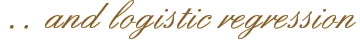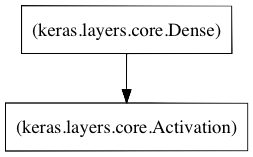##A follow-up on the collaborative filter, it occurs to me that it should be possible to add a logistic regression layer in Keras.  The following is a regularized multinomial logistic regression model:

```from keras.models import Sequential
from keras.layers.core import Dense, Activation
from keras.regularizers import l2

model = Sequential()